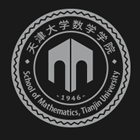﻿A Globally and Quadratically Convergent Algorithm for Solving Multilinear Systems with M-tensors-天津大学数学学院

# A Globally and Quadratically Convergent Algorithm for Solving Multilinear Systems with M-tensors

2019-10-09 11:04

We consider multilinear systems of equations whose coefficient tensors areMtensors. Multilinear systems of equations have many applications in engineering and scientific computing, such as data mining and numerical partial differential equations. In this talk, we show that solving multilinear systems withM-tensors is equivalent to solving nonlinear systems of equations where the involving functions are P-functions. Based on this result, we propose a Newton-type method to solve multilinear systems withM-tensors. For a multilinear system with a nonsingularM-tensor and a positive right side vector, we prove that the sequence generated by the proposed method converges to the unique solution of the multilinear system and the convergence rate is quadratic. Numerical results are reported to show that the proposed method is promising.

This is a joint work with H. J. He, L. Q. Qi and G.L. Zhou.### 联系我们